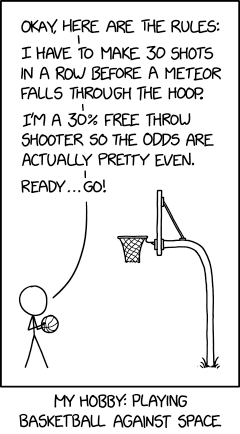The subreddit r/TheyDidTheMath has a bunch of useless math calculations for real world “applications.” It’s quite similar to Fermi Questions, my favorite Science Olympiad event. Last night, I saw an xkcd that made me want to check it’s math.Let’s see if this math is correct. (I don’t have any doubt, but I’m bored so here we go!)

## Checking the math - basketball

Okay, so 30 shots in a row at a 30% accuracy would be: $$(.3)^{30} = 2.06*10^{-16}$$

I’m only using 3 significant figures for this to keep things short. (Also because it doesn’t really matter.)

## Checking the math - space

The next part is a little bit harder: what is the probability that a meteor travels through a basketball hoop-sized hole?

Actually, there’s a small distinction to make: meteors are the space rocks that fly through earth’s atmosphere, while meteorites are meteors that survive and hit the ground. In this case, I’ll be looking at statistics for meteorites that are larger than 1 inch in diameter so that it’s actually possible to verify that the space rock exists. If you can’t see it, then space didn’t win yet.

Unfortunately, nobody keeps track of meteroites because they’re super frequent and vary greatly in size. Literally every single website I’ve stumbled across has a different value. For this, I’ll be using an estimate of 500 noticable meteorites on earth per year. I know that there’s a lot of factors that determine the probability of a meteor falling in an exact spot (location, weather, etc.) but this will have to do. Now, we just need a few more values:

• Earth’s surface area: 197 million square miles
• Basketball hoop diameter: 18 inches

$$(18/2)^2*\pi = 254 \text{ in}^2$$

• Basketball hoop area: 254 square inches

$$\frac{254}{5280^2 * 12^2 * 197*10^6} = 3.21 * 10^{-16}$$

Dang, comparing that value to the earlier value is super close! However, there’s still one thing I want to check: time.

## Time

Let’s assume that you shoot free throws non-stop (24/7/365) until you make 30 in a row (at a constant 30% accuracy). Let’s also assume that you shoot a ball every 10 seconds since nobody wants to retrieve balls for you.

$$\frac{1}{2.06 * 10^{-16}} = 4.86 * 10^{15}$$

$$\frac{4.86*10^{15}} {6 * 3600 * 24 * 365} = 25668737 \text{ years}$$

It will take over 25 million years to make 30 free throws in a row. Now let’s take our 500 meteorites per year and see how long it’ll take for space to make a shot.

$$\frac{1}{3.21 * 10^{-16} * 500} = 6.22 * 10^{12} \text{ years}$$

Okay, that’s actually quite a large difference. It will take over 242 thousand times as long for space to make a shot than for you to make 30 in a row at 30% accuracy, assuming an attempt every 10 seconds for thr next 25 million years.

## Conclusion

Of course, there’s a lot of possible error from many steps - changing the throw frequency, meteoroid size, and meteoroid frequency will all affect the end result drastically. Here’s the code for a calculator to see how many shots to make in a row for a fair 1v1 against space (using 6 trillion years for space to make a shot). Just run it with Python and it should work.

import math

accuracy = float(input("Enter your accuracy here as a decimal:"))

permin = float(input("Enter your attempts per minute here:"))

shots = str(math.ceil((math.log(5.285*10**(-21)) - math.log(permin)) / math.log(accuracy))) # I know it's bad code sorry but it works if you don't try to break it lol

print("You need to make " + shots + " shots in a row. Have fun.")


Fun fact: I wrote this entire post (including the calculator) on my iPad without spellcheck or preview, so if there are typos, that is why.